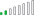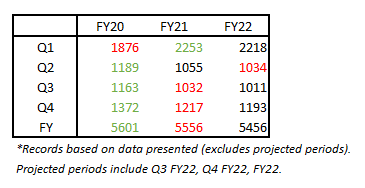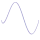cancel
Showing results for
Did you mean:Resolver II

## Conditional Formatting with DAX

Hi,

I am attempting to use a calculated measure to determine some conditional formatting in a matrix. I have attached sample data.

In this data, I have two separate versions of data: Actual and Projection. In the matrix, I have used a calculated measure so that for completed period, the "Actual" version will show. But for projected periods (Q3 FY22, Q4 FY22, and yearly FY22 values), the "Projection" version will show. This has been achieved with the following measure:

``````Sales_ =
SWITCH(
TRUE(),
CALCULATE(
SUM('Data Table'[Sales]),
FILTER('Data Table', 'Data Table'[Version] = "Actual")) =
CALCULATE(
SUM('Data Table'[Sales]),
FILTER('Data Table', 'Data Table'[Version] = "Projection")),
CALCULATE(
MAX('Data Table'[Sales]),
FILTER('Data Table', 'Data Table'[Version] = "Actual")),
CALCULATE(
MAX('Data Table'[Sales]),
FILTER('Data Table', 'Data Table'[Version] = "Current Projection")
))``````

Now, we move on to the conditional formatting. First rule: I need the conditional formatting to exclude projected periods (Q3 FY22, Q4 FY22, and yearly FY22). The text here will remain at defaulted black. Next, I want the maximum value for each row (quarter) to be in green text and the minimum value for each row (quarter) to be in red text. So, although each of the projected periods have lowers values than any other years, these projected values must remain in black text, and the next smallest number will be in red text, indicating minimum values. I have achieved this to an extent with the following measure:

``````Sales Conditional Formatting =
VAR Total = CALCULATE(SELECTEDVALUE('Data Table'[Sales]), ALLSELECTED('Data Table'[Year]), FILTER('Data Table', 'Data Table'[Sales] <> 0), FILTER('Data Table', 'Data Table'[Version] = "Actual"))
VAR MaxTotal = CALCULATE(MAX('Data Table'[Sales]), ALLSELECTED('Data Table'[Year]), KEEPFILTERS('Data Table'[Version] = "Actual"))
VAR MinTotal = CALCULATE(MIN('Data Table'[Sales]), ALLSELECTED('Data Table'[Year]), KEEPFILTERS('Data Table'[Sales] <> 0), KEEPFILTERS('Data Table'[Version] = "Actual"))
return
SWITCH(
TRUE(),
Total = MaxTotal, "#007A00",
Total = MinTotal, "#FF0000")``````

I have achieved this as far as the actual quarters are concerned. When the "Actual" value is zero like it is in Q3 FY22 and Q4 FY22, these periods will be excluded, so Q3 FY21 and Q4 FY21 will be in red text. This is exactly how I need it. However, for the yearly values row, it is still reading the yearly FY22 value as the minimum. In the calculated measure above, it is actually reading the "Actual" value (which is 2,863 - the running total of "Actual" values for the year, Q1 FY22 + Q2 FY22). If I change the VAR MinTotal above to this:

VAR MinTotal = CALCULATE(MIN('Data Table'[Sales]), ALLSELECTED('Data Table'[Year]), KEEPFILTERS('Data Table'[Sales] <> 0), KEEPFILTERS('Data Table'[Version] = "Projection")), it does not show red text for Q3, Q4, or yearly rows. I assume this is because the "Projected" value is the minimum, but we are excluded "Projected" values from formatting, earlier in the measure. If I change the VAR MinTotal above to this:

VAR MinTotal = CALCULATE(MIN('Data Table'[Sales]), ALLSELECTED('Data Table'[Year]), KEEPFILTERS('Data Table'[Sales] <> 0), FILTER('Data Table', 'Data Table'[Version] = "Projection")), it changes all values to red text except what is in green and the excluded projected periods which are in black.

See image below for desired output. View the sample data here. (google docs) Thanks!1 ACCEPTED SOLUTIONCommunity Support

Hi @amconnel ,

Let the min in red and max in green, and if "Actual" value is zero, then this period in red, which result basd on [sales_] of each rows.

Please try this code to do that:

``````Measured =
VAR _s =
FILTER (
SUMMARIZE (
ALLSELECTED ( 'Data Table'[Year] ),
[Year],
"Sale",
IF (
COUNTROWS (
FILTER ( 'Data Table', 'Data Table'[Version] = "Actual" && [Sales] = 0 )
) > 0,
BLANK (),
[Sales_]
)
),
[Sale] > 0
)
VAR _ss =
FILTER (
SUMMARIZE (
CALCULATETABLE (
'Data Table',
ALLSELECTED ( 'Data Table'[Year] ),
'Data Table'[Quarter] <> "FY"
),
[Year],
"Sale",
IF (
COUNTROWS (
FILTER ( 'Data Table', 'Data Table'[Version] = "Actual" && [Sales] = 0 )
) > 1,
0,
[Sales_]
)
),
[Sale] > 0
)
VAR _max =
IF (
SELECTEDVALUE ( 'Data Table'[Quarter] ) = "FY",
MAXX ( TOPN ( 1, _ss, [Sale], DESC ), [Year] ),
MAXX ( TOPN ( 1, _s, [Sale], DESC ), [Year] )
)
VAR _min =
IF (
SELECTEDVALUE ( 'Data Table'[Quarter] ) = "FY",
MAXX ( TOPN ( 1, _ss, [Sale], ASC ), [Year] ),
MAXX ( TOPN ( 1, _s, [Sale], ASC ), [Year] )
)
RETURN
SWITCH (
SELECTEDVALUE ( 'Data Table'[Year] ),
_max, "green",
_min, "red",
"black"
)
``````

Result:Pbix in the end you can refer.

Best Regards

Community Support Team _ chenwu zhu

If this post helps, then please consider Accept it as the solution to help the other members find it more quickly.

3 REPLIES 3Community Support

Hi @amconnel ,

Let the min in red and max in green, and if "Actual" value is zero, then this period in red, which result basd on [sales_] of each rows.

Please try this code to do that:

``````Measured =
VAR _s =
FILTER (
SUMMARIZE (
ALLSELECTED ( 'Data Table'[Year] ),
[Year],
"Sale",
IF (
COUNTROWS (
FILTER ( 'Data Table', 'Data Table'[Version] = "Actual" && [Sales] = 0 )
) > 0,
BLANK (),
[Sales_]
)
),
[Sale] > 0
)
VAR _ss =
FILTER (
SUMMARIZE (
CALCULATETABLE (
'Data Table',
ALLSELECTED ( 'Data Table'[Year] ),
'Data Table'[Quarter] <> "FY"
),
[Year],
"Sale",
IF (
COUNTROWS (
FILTER ( 'Data Table', 'Data Table'[Version] = "Actual" && [Sales] = 0 )
) > 1,
0,
[Sales_]
)
),
[Sale] > 0
)
VAR _max =
IF (
SELECTEDVALUE ( 'Data Table'[Quarter] ) = "FY",
MAXX ( TOPN ( 1, _ss, [Sale], DESC ), [Year] ),
MAXX ( TOPN ( 1, _s, [Sale], DESC ), [Year] )
)
VAR _min =
IF (
SELECTEDVALUE ( 'Data Table'[Quarter] ) = "FY",
MAXX ( TOPN ( 1, _ss, [Sale], ASC ), [Year] ),
MAXX ( TOPN ( 1, _s, [Sale], ASC ), [Year] )
)
RETURN
SWITCH (
SELECTEDVALUE ( 'Data Table'[Year] ),
_max, "green",
_min, "red",
"black"
)
``````

Result:Pbix in the end you can refer.

Best Regards

Community Support Team _ chenwu zhu

If this post helps, then please consider Accept it as the solution to help the other members find it more quickly.Resolver II

I was finally able to make this work, so thank you!! I had to make a couple of changes for some formatting differences between my file and the sample I shared but finally got it to work.

Is there any reason a similar measure should not work for a metric that is coming from a calculated column? Sales and Volume are an original part of my data source. I also want to view sales per pound, so I created a calculated column for this new metric. I am wanting to show the same type of conditional formatting for this metric, but I cannot get FY22 to be excluded from the formatting. The quarters are formatting correctly.Resolver II

I was able to fix it. I needed to insert an additional filter in the DAX for the calculated measure for the conditional formatting measure to work.

All problems are solved. Thanks so much for your help!!